# Name Have I learned This – Alg2/Trig Unit 5 Test Graph and Solve Polynomial Functions Parent Signature sol: t1, t4, t7, t8 Teacher Signature Date of Retake Complete the statement to describe the end behavior of the graph of the function

 Name__________________________________ Have I Learned This – Alg2/Trig Unit 5 Test Graph and Solve Polynomial Functions Parent Signature_________________________ SOL: A2.T1, A2.T4, A2.T7, A2.T8 Teacher Signature________________________ Date of Retake___________________ Complete the statement to describe the end behavior of the graph of the function. 1.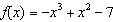2.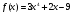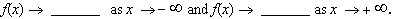Write a polynomial function f of least degree that has rational coefficients, a leading coefficient of 1, and the given zeros. 3. 4. –3, 0, 5 5.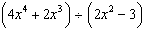6. Give an example of a cubic function which has a 7. Divide. graph that intersects the x-axis in exactly two points. 8. Two zeros of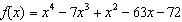are and 8. a. How many additional zeros are there? Explain. b. How many turning points does the graph of f have? Explain. c. Find all local maximums and local minimums. Then find the range of the function.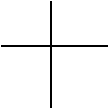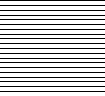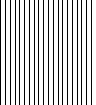9. Using the polynomial function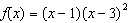, a. Explain what effect the exponent of each factor has on the graph of the polynomial. b. Make a sketch of the graph. 10. Evaluate the polynomial function when d = 4: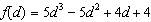Find the real-number solutions of the equation. 11. 12.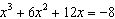Factor the polynomial completely. 13. 14. 15. 16.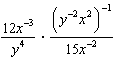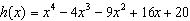17. Simplify the expression. 18. Find the real zeros.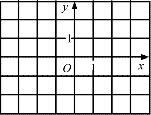19. Sketch the graph of a third-degree polynomial function that has one local maximum, one local minimum, and three zeros. Identify each of these points. 20. Evaluate: 21. List the possible rational zeros of the function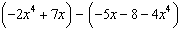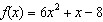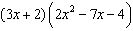22. Write the cubic function whose graph is shown. 23. Find the product: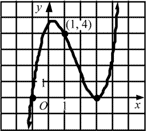Find all zeros of the polynomial function. 24.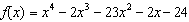25.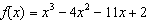26.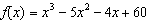27. Graph the polynomial function. 28. Use the graph to approximate the zeros of the function.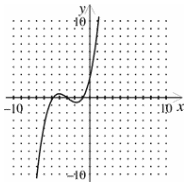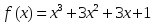29. Write a cubic function who’s graph passes through the points , , , and ? 30. A catering company is designing a box for packing chocolate covered nuts. The company would like the volume of the box to be 54 cubic inches and the bottom of the box to be a square. Suppose the bottom of the box has a width that is 3 inches smaller than the height x of the box. Download 152.05 Kb.Share with your friends: